Next: The mean, variance, and Up: Probability theory Previous: Combinatorial analysis

## The binomial distribution

It follows from Eqs. (16) and (20) that the probability of obtaining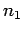occurrences of the outcome 1 instatistically independent observations of a two-state system is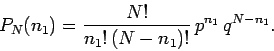(21)

This probability function is called the binomial distribution function. The reason for this is obvious if we tabulate the probabilities for the first few possible values of(see Tab. 1).

Table 1: The binomial probability distribution0 1 2 3 4 1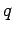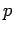2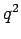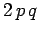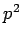3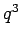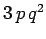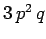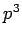4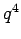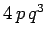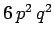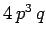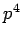Of course, we immediately recognize these expressions: they appear in the standard algebraic expansions of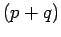,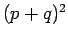,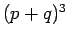, and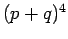, respectively. In algebra, the expansion of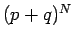is called the binomial expansion (hence, the name given to the probability distribution function), and can be written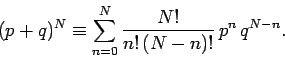(22)

Equations (21) and (22) can be used to establish the normalization condition for the binomial distribution function: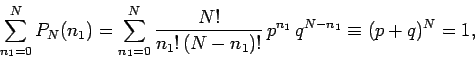(23)

since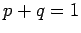.Next: The mean, variance, and Up: Probability theory Previous: Combinatorial analysis
Richard Fitzpatrick 2006-02-02